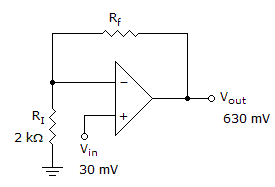# Electronics - Operational Amplifiers

### Exercise :: Operational Amplifiers - Filling the Blanks

16.The voltage gain of the amplifier in the given circuit would increase if ______.

 A. the feedback resistor was decreased in value B. the input voltage was increased C. the input resistor was increased in value D. None of the above

Explanation:

No answer description available for this question. Let us discuss.

17.

A virtual ground is a(n) ______ ground because this point is at zero volts.

 A. ordinary B. voltage C. chassis D. earth

Explanation:

No answer description available for this question. Let us discuss.

18.

The resistors Rf and Ri in an op-amp circuit control ______ .

 A. positive feedback and current gain B. negative feedback and power gain C. negative feedback and voltage gain D. open and closed loop gain

Explanation:

No answer description available for this question. Let us discuss.

19.

______ is NOT a characteristic of an ideal op-amp.

 A. Infinite voltage gain B. Minimum bandwidth C. Infinite input impedance D. Zero output impedance

Explanation:

No answer description available for this question. Let us discuss.

20.

Current cannot flow to ground through a(n) ________.

 A. mechanical ground B. ac ground C. virtual ground D. virtual short